# Formula and Equations For Inductor and Inductance

## Inductor and Inductance Formulas and Equations

The following formulas and equations can be used to calculate the inductance and related quantities of different shapes of inductors as follow.### Inductance of Inductor:

The inductance of the inductor from the basic formula of inductor:#### Voltage across Inductor: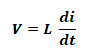#### Current of the Inductor: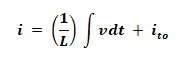Where

• V is the voltage across inductor
• L is the inductance of the inductor in Henry
• Di/dt is the instantaneous rate of current change through the inductor.
• ito = current at time t = 0.

#### Reactance of the Inductor:

Inductive reactance is the opposition of inductor to alternating current AC, which depends on its frequency f and is measured in Ohm just like resistance. Inductive reactance is calculated using:

X= ωL = 2πfL

Where

• Xis the Inductive reactance
• f is the applied frequency
• L is the Inductance in Henry

#### Quality Factor of Inductor:

The efficiency of the inductor is known as quality factor & its measured by:

QF = XL/ESR

Where

• Xis the Inductive reactance
• ESR is the equivalent series resistance of the circuit.

#### Dissipation Factor of Inductor:

It is the inverse of the quality factor and it shows the power dissipation inside the inductor & its given by:

DF = tan δ = ESR/XL

Where

• DF is the dissipation factor
• δ is the angle between capacitive reactance victor & negative axis.
• Xis the capacitive reactance
• ESR is the equivalent series resistance of the circuit.

### Energy Stored in an Inductor:

The energy E stored in inductor is given by:

E = ½ Li2

Where

• E is the energy in joules
• L is the inductance in Henry
• i is the current in Amps

Related Posts:

#### Average Power of Inductor

The average power for the inductor is given by:

Pav = Li2 / 2t

Where

• = is the time in seconds.

### Inductor Current During Charge / Discharge:

Just like capacitor, the inductor takes up to 5 time constant to fully charge or discharge, during this time the current can be calculated by:

#### During Charging:

Instantaneous current of the inductor during charging is given by:#### During Discharging:

The current during the discharging at any time t is given by:Where

• IC is the current of the inductor
• I0 is the current at time t=0
• t  is the time passed after supplying current.
• τ = L/R is the time constant of the RL circuit

Related Posts:

### Inductance Formulas

#### Helical Coil Inductance “Wheeler’s Formula”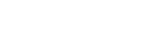Where:

• L is the inductance
• n is the number of turns
• h is the height

#### Spiral Coil Inductance Formula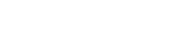Where:

• OR is the outermost radius in inches
• IR is the innermost radius in inches

#### Spiral Coil Wire Length Formula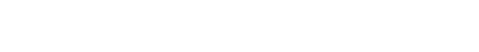#### Conical Coil Inductance Formula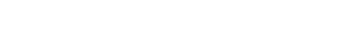Where:

• θ is the angle outside the cone, (assume θ ≈ 15°)

Related Formulas and Equations Posts: